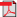RIEB Discussion Paper Series No.2015-11Title

Fast Bellman Iteration: An Application of Legendre-Fenchel Duality to Infinite-Horizon Dynamic Programming in Discrete Time

Abstract

We propose an algorithm, which we call "Fast Bellman Iteration" (FBI), to compute the value function of an infinite-horizon dynamic programming problem in discrete time. FBI is an extremely efficient linear-time algorithm applicable to a class of multidimensional dynamic programming problems with concave return (or convex cost) functions and linear constraints. In this algorithm, a sequence of functions is generated starting from the zero function by repeatedly applying a simple algebraic rule involving the Legendre-Fenchel transform of the return function. The resulting sequence is guaranteed to converge, and the Legendre-Fenchel transform of the limiting function coincides with the value function.

Ronaldo CARPIO